# Site map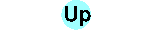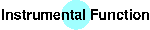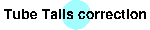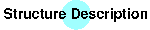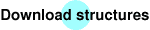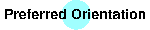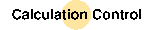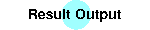## BGMN Control File

The Rietveld calculation with BGMN is controlled by a simple text file with the file extension 'sav'.

By means of the control file for the plaster sample (`plaster.sav`) the necessary elements will be explained here. Please open BGMNwin and select
File→Open Control File,
browse to the PLASTER\plaster.sav file and open it: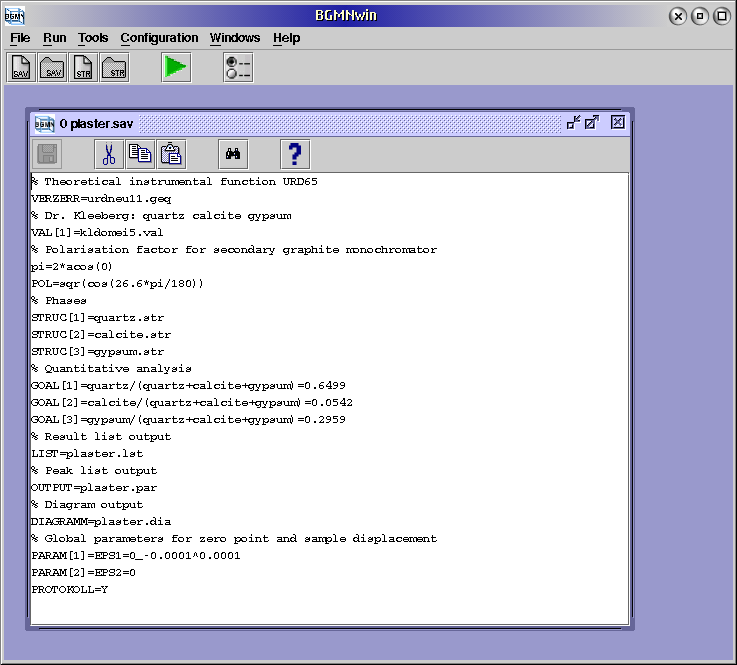For an extended explanation of a variable put the cursor of your BGMNwin window to that variable and press F1 or see a list of variables.

First, the instrumental function has to be provided:

```VERZERR=urdneu11.geq
```
Then the pattern data file is needed:
```VAL=kldomei5.val
```

This file is in the APX-63 format, a simple text format from the SEIFERT-FPM diffractometers. Also, BGMN can process files with the extension 'rd' (Philips APD) and 'raw' (SIEMENS Diffrac-AT) and two variants of 'gsa' (GSAS) as well. If you want to use other formats, you have to convert it into one of the above.

If a secondary graphite monochromator was used, an appropriate polarisation factor is (26.6° is the 2Θ angle of the graphite crystal; without monochromator use 'POL=1', which is the default):

```pi=2*acos(0)
POL=sqr(cos(26.6*pi/180))
```
The structure files are given in this way:
```STRUC=quartz.str
STRUC=calcite.str
STRUC=gypsum.str
```
And the formulae for the quantitative analysis are:
```GOAL=quartz/(quartz+calcite+gypsum)
GOAL=calcite/(quartz+calcite+gypsum)
GOAL=gypsum/(quartz+calcite+gypsum)
```
In this file (`plaster.lst`) the results are written:
```LIST=plaster.lst
```
An output file for the peak list (`plaster.par`) is necessary if you want a peak list:
```OUTPUT=plaster.par
```
For the easy inspection of diagrams a file (`plaster.dia`) with pattern, calculated, difference and background data can be written:
```DIAGRAMM=plaster.dia
```
Last, the global parameters for the zero point (`EPS1`) and sample displacement (`EPS2`) are included:
```PARAM=EPS1=0_-0.0001^0.0001
PARAM=EPS2=0
```
Select
```PROTOKOLL=Y
```

If you made any changes to plaster.sav, please save it. The calculation is started by the command
Run→BGMN
Browse to the `plaster.sav` file and open it, and after each iteration step a line with information about the iteration number, the least squares sum and some other values is printed.

## Structure Output Files

If one wants to inspect/process the resulting structure(s), three structure formats can be written:
• ShelX output: `RESOUT=quartz.res`
This format can be processed among others by the program PowderCell.

• PDB output: `PDBOUT=quartz.pdb`
(used with RasMol)

• BGMN output: ` STRUCOUT=quartz.sto`
(for input in further BGMN calculations)
The above mentioned statements have to be included in the BGMN control file for each structure.

For further output see Result Output